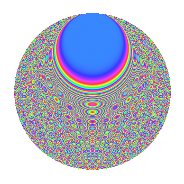# Properties

 Label 3024.2.duLevel 3024 Weight 2 Character orbit du Rep. character $$\chi_{3024}(341,\cdot)$$ Character field $$\Q(\zeta_{12})$$ Dimension 752 Sturm bound 1152

# Related objects

## Defining parameters

 Level: $$N$$ = $$3024 = 2^{4} \cdot 3^{3} \cdot 7$$ Weight: $$k$$ = $$2$$ Character orbit: $$[\chi]$$ = 3024.du (of order $$12$$ and degree $$4$$) Character conductor: $$\operatorname{cond}(\chi)$$ = $$1008$$ Character field: $$\Q(\zeta_{12})$$ Sturm bound: $$1152$$

## Dimensions

The following table gives the dimensions of various subspaces of $$M_{2}(3024, [\chi])$$.

Total New Old
Modular forms 2352 784 1568
Cusp forms 2256 752 1504
Eisenstein series 96 32 64

## Trace form

 $$752q - 4q^{4} + 6q^{5} + O(q^{10})$$ $$752q - 4q^{4} + 6q^{5} - 12q^{10} + 6q^{11} + 6q^{14} - 4q^{16} - 12q^{19} - 4q^{22} + 12q^{29} + 12q^{34} + 30q^{35} - 4q^{37} + 6q^{38} - 6q^{40} - 4q^{43} + 66q^{44} - 12q^{46} + 24q^{47} - 4q^{49} + 12q^{50} - 6q^{52} + 48q^{56} + 2q^{58} + 12q^{59} - 24q^{62} - 16q^{64} - 4q^{67} + 6q^{68} + 26q^{70} - 78q^{74} + 6q^{77} - 8q^{79} - 24q^{82} - 60q^{83} + 6q^{85} + 66q^{86} + 2q^{88} + 20q^{91} + 12q^{92} + 90q^{98} + O(q^{100})$$

## Decomposition of $$S_{2}^{\mathrm{new}}(3024, [\chi])$$ into newform subspaces

The newforms in this space have not yet been added to the LMFDB.

## Decomposition of $$S_{2}^{\mathrm{old}}(3024, [\chi])$$ into lower level spaces

$$S_{2}^{\mathrm{old}}(3024, [\chi]) \cong$$ $$S_{2}^{\mathrm{new}}(1008, [\chi])$$$$^{\oplus 2}$$

## Hecke Characteristic Polynomials

There are no characteristic polynomials of Hecke operators in the database